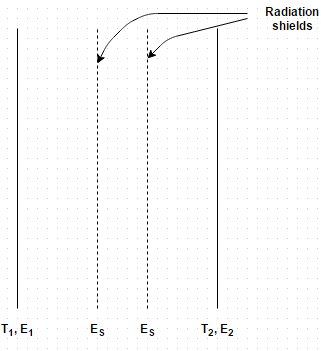## 10 Questions MCQ Test Heat Transfer for Engg. | Test: Radiations Shields

Description
This mock test of Test: Radiations Shields for Chemical Engineering helps you for every Chemical Engineering entrance exam. This contains 10 Multiple Choice Questions for Chemical Engineering Test: Radiations Shields (mcq) to study with solutions a complete question bank. The solved questions answers in this Test: Radiations Shields quiz give you a good mix of easy questions and tough questions. Chemical Engineering students definitely take this Test: Radiations Shields exercise for a better result in the exam. You can find other Test: Radiations Shields extra questions, long questions & short questions for Chemical Engineering on EduRev as well by searching above.
QUESTION: 1

Solution:

Reflexive power is much high for radiation shield.

QUESTION: 2

### Radiation shield are used between the emitting surfaces such that

Solution:

Many situations are encountered where it is desired to reduce the overall heat transfer between two radiating surfaces.

QUESTION: 3

### Which of the following can be used as a radiating shield?

Solution:

The shields are thin opaque partitions arranged in the direction perpendicular to the propagation of radiant heat.

QUESTION: 4

Two large parallel planes with emissivity 0.4 are maintained at different temperatures and exchange heat only by radiation. What percentage change in net radiative heat transfer would occur if two equally large radiation shields with surface emissivity 0.04 are introduced in parallel to the plates?Solution:

When shields are not used, Q 12 = (F g) 12 A 1 σ b (T 14 – T 24) = 0.2 A 1 σ b (T 14– T 24) and when shields are used Q 12 = 0.0098 A 1 σ b (T 14 – T 24).

QUESTION: 5

Determine the net radiant heat exchange per m 2 area for two infinite parallel plates held at temperature of 800 K and 500 K. Take emissivity as 0.6 for the hot plate and 0.4 for the cold plate

Solution:

12 = (F g) 12 A 1 σ b (T 14 – T 24) and (F g) 12 = 0.135. Therefore, Q 12 = 6200 W/m2.

QUESTION: 6

Consider the above problem, what should be the emissivity of a polished aluminum shield placed between them if heat flow is to be reduced to 40 percent of its original value?

Solution:

(Fg) 12 = 1/E 1 +1/E 2 +2/E 3 – 2 = 7.936.

QUESTION: 7

Consider radiative heat transfer between two large parallel planes of surface emissivities 0.8. How many thin radiation shields of emissivity 0.05 be placed between the surfaces is to reduce the radiation heat transfer by a factor of 75?

Solution:

(Q 12ONE SHIELD = A σ b (T 14 – T 24)/ 1/E 1 +1/E 2 +2/E 3 – 2 and 75 = (Q 12NO SHIELD / (Q 12N SHIELD.

QUESTION: 8

Two parallel square plates, each 4 m2 area, are large compared to a gap of 5 mm separating them. One plate has a temperature of 800 K and surface emissivity of 0.6, while the other has a temperature of 300 K and surface emissivity of 0.9. Find the net energy exchange by radiations between the plates

Solution:

12 = (F g) 12 A 1 σ b (T 14 – T 24).

QUESTION: 9

The furnace of a boiler is laid from fire clay brick with outside lagging from the plate steel, the distance between the two is quite small compared with the size of the furnace. The brick setting is at an average temperature of 365 K whilst the steel lagging is at 290 K. Calculate the radiant heat flux. Assume the following emissivity values
For brick = 0.85
For steel = 0.65

Solution:

12 = (F g) 12 A 1 σ b (T 14 – T 24).

QUESTION: 10

Consider the above problem, find the reduction in heat loss if a steel screen having an emissivity value of 0.6 on both sides is placed between the brick and steel setting

Solution:

(F g) 12 = 0.247 and Q = 149.51 W/m2.